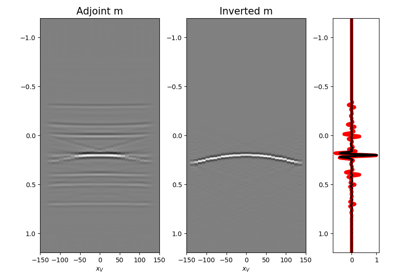# pylops.waveeqprocessing.MDD¶

pylops.waveeqprocessing.MDD(G, d, dt=0.004, dr=1.0, nfmax=None, wav=None, twosided=True, causality_precond=False, adjoint=False, psf=False, dtype='float64', dottest=False, saveGt=True, add_negative=True, **kwargs_lsqr)[source]

Multi-dimensional deconvolution.

Solve multi-dimensional deconvolution problem using scipy.sparse.linalg.lsqr iterative solver.

Parameters: G : numpy.ndarray Multi-dimensional convolution kernel in time domain of size $$[n_s \times n_r \times n_t]$$ for twosided=False or twosided=True and add_negative=True (with only positive times) or size $$[n_s \times n_r \times 2*n_t-1]$$ for twosided=True and add_negative=False (with both positive and negative times) d : numpy.ndarray Data in time domain $$[n_s (\times n_vs) \times n_t]$$ dt : float, optional Sampling of time integration axis dr : float, optional Sampling of receiver integration axis nfmax : int, optional Index of max frequency to include in deconvolution process wav : numpy.ndarray, optional Wavelet to convolve to the inverted model and psf. If None, the outputs of the inversion are returned directly twosided : bool, optional MDC operator and data both negative and positive time (True) or only positive (False) add_negative : bool, optional Add negative side to MDC operator and data (True) or not (False)- operator and data are already provided with both positive and negative sides. To be used only with twosided=True. causality_precond : bool, optional Apply causality mask (True) or not (False) adjoint : bool, optional Compute and return adjoint(s) psf : bool, optional Compute and return Point Spread Function (PSF) and its inverse dtype : bool, optional Type of elements in input array. dottest : bool, optional Apply dot-test saveGt : bool, optional Save G and G^H to speed up the computation of adjoint of pylops.signalprocessing.Fredholm1 (True) or create G^H on-the-fly (False) Note that saveGt=True will be faster but double the amount of required memory **kwargs_lsqr Arbitrary keyword arguments for scipy.sparse.linalg.lsqr solver minv : numpy.ndarray Inverted model of size $$[n_r (\times n_{vs}) \times n_t]$$ for twosided=False or $$[n_r (\times n_vs) \times 2*n_t-1]$$ for twosided=True madj : numpy.ndarray Adjoint model of size $$[n_r (\times n_{vs}) \times n_t]$$ for twosided=False or $$[n_r (\times n_r) \times 2*n_t-1]$$ for twosided=True psfinv : numpy.ndarray Inverted psf of size $$[n_r \times n_r \times n_t]$$ for twosided=False or $$[n_r \times n_r \times 2*n_t-1]$$ for twosided=True psfadj : numpy.ndarray Adjoint psf of size $$[n_r \times n_r \times n_t]$$ for twosided=False or $$[n_r \times n_r \times 2*n_t-1]$$ for twosided=True

MDC
Multi-dimensional convolution

Notes

Multi-dimensional deconvolution (MDD) is a mathematical ill-solved problem, well-known in the image processing and geophysical community .

MDD aims at removing the effects of a Multi-dimensional Convolution (MDC) kernel or the so-called blurring operator or point-spread function (PSF) from a given data. It can be written as

$\mathbf{d}= \mathbf{D} \mathbf{m}$

or, equivalently, by means of its normal equation

$\mathbf{m}= (\mathbf{D}^H\mathbf{D})^{-1} \mathbf{D}^H\mathbf{d}$

where $$\mathbf{D}^H\mathbf{D}$$ is the PSF.

  Wapenaar, K., van der Neut, J., Ruigrok, E., Draganov, D., Hunziker, J., Slob, E., Thorbecke, J., and Snieder, R., “Seismic interferometry by crosscorrelation and by multi-dimensional deconvolution: a systematic comparison”, Geophyscial Journal International, vol. 185, pp. 1335-1364. 2011.

## Examples using pylops.waveeqprocessing.MDD¶08. Multi-Dimensional Deconvolution